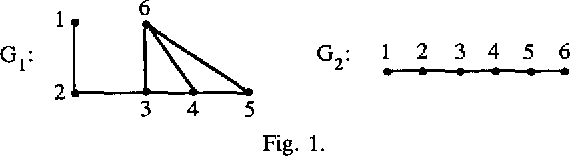You are currently offline. Some features of the site may not work correctly.

# Erdős–Gallai theorem

Known as: Erdos-Gallai theorem, Erdos–Gallai theorem, Erdős-Gallai theorem
The Erdős–Gallai theorem is a result in graph theory, a branch of combinatorial mathematics. It provides one of two known approaches solving the… Expand
Wikipedia

## Papers overview

Semantic Scholar uses AI to extract papers important to this topic.
2020
2020
• 2020
• Corpus ID: 52063737
In this paper, we prove a theorem on tight paths in convex geometric hypergraphs, which is asymptotically sharp in infinitely… Expand
•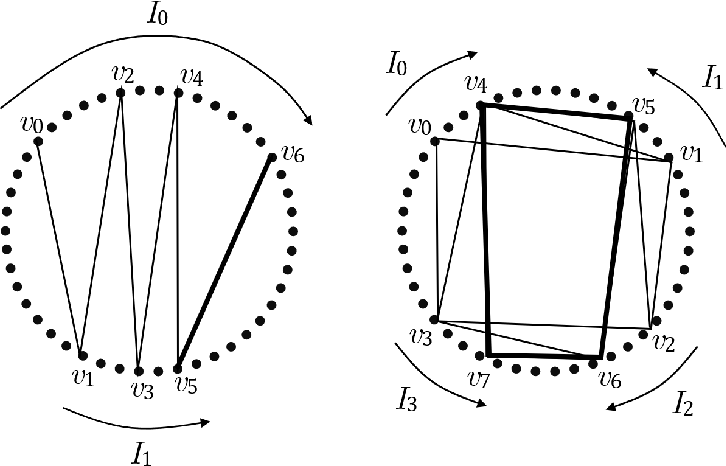•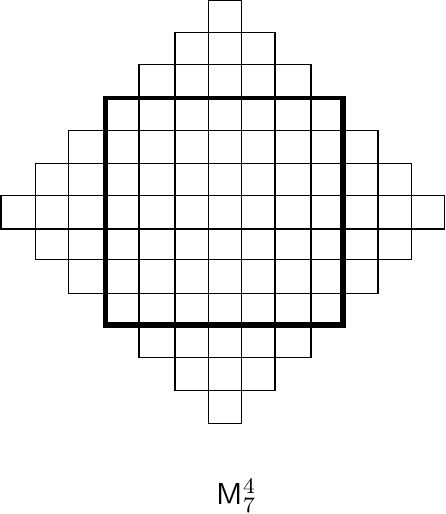•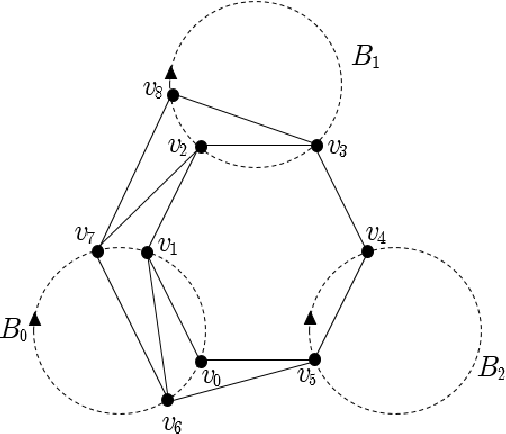2019
2019
• Discret. Math.
• 2019
• Corpus ID: 59336609
Abstract The Erdős–Gallai Theorem states that every graph of average degree more than l − 2 contains a path of order l for l ≥ 2… Expand
2018
2018
• Discret. Math.
• 2018
• Corpus ID: 4606111
Abstract The Erdős–Gallai Theorem states that for k ≥ 3 , any n -vertex graph with no cycle of length at least k has at most 1 2… Expand
2017
2017
• 2017
• Corpus ID: 119579444
The Erd\H{o}s--Gallai Theorem states that for \$k \geq 3\$, any \$n\$-vertex graph with no cycle of length at least \$k\$ has at most… Expand
•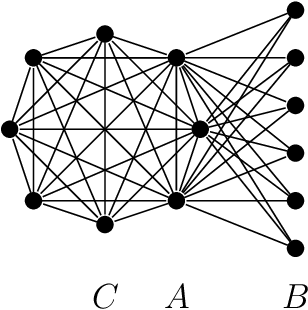•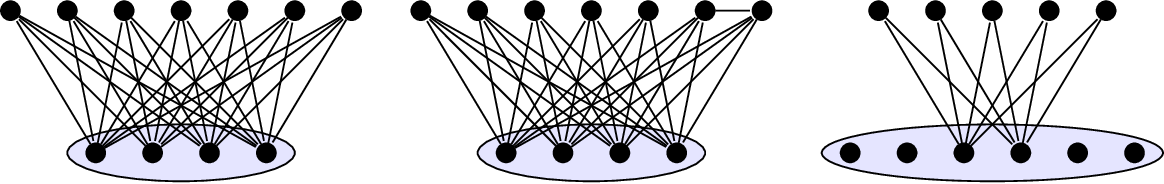•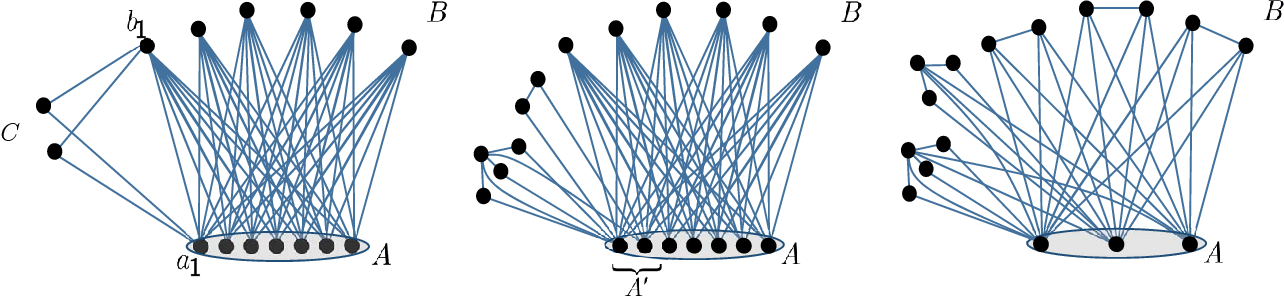•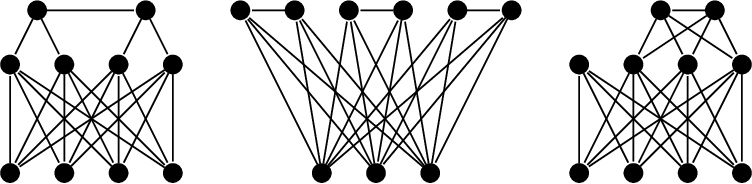2016
2016
• Eur. J. Comb.
• 2016
• Corpus ID: 38506536
We extend the Erdźs-Gallai Theorem for Berge paths in r -uniform hypergraphs. We also find the extremal hypergraphs avoiding t… Expand
2016
2016
• J. Comb. Theory, Ser. B
• 2016
• Corpus ID: 205930688
The Erd\H{o}s-Gallai Theorem states that for \$k \geq 2\$, every graph of average degree more than \$k - 2\$ contains a \$k\$-vertex… Expand
•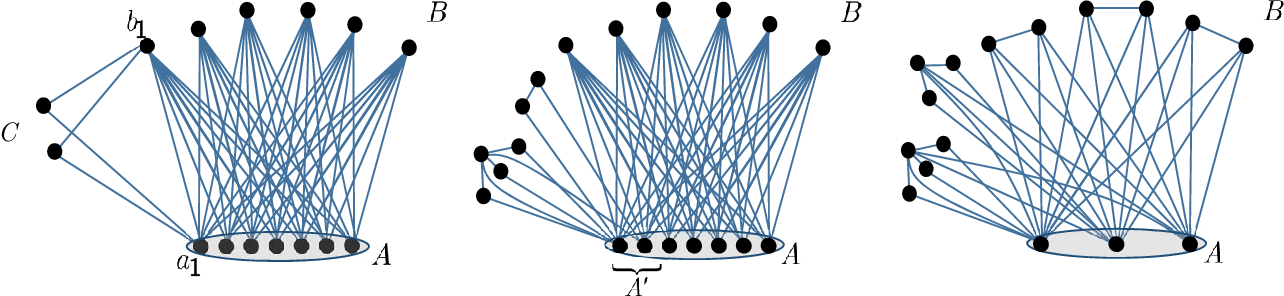2010
2010
• Electron. Notes Discret. Math.
• 2010
• Corpus ID: 13214259
Abstract The Erdős-Gallai Theorem gives the maximum number of edges in a graph without a path of length k. We extend this result… Expand
2008
2008
If m is a positive integer then we call a tree on at least 2 vertices an m-tree if no vertex is adjacent to more than m leaves… Expand
2007
2007
• Discret. Math.
• 2007
• Corpus ID: 28194744
A classical result on extremal graph theory is the Erdos-Gallai theorem: if a graph on n vertices has more than (k-1)n2 edges… Expand
1988
1988
• Discret. Math.
• 1988
• Corpus ID: 10684516
Publisher Summary In 1959 Gallai presented his now classical theorem, involving the vertex covering number α 0 , the vertex… Expand
•